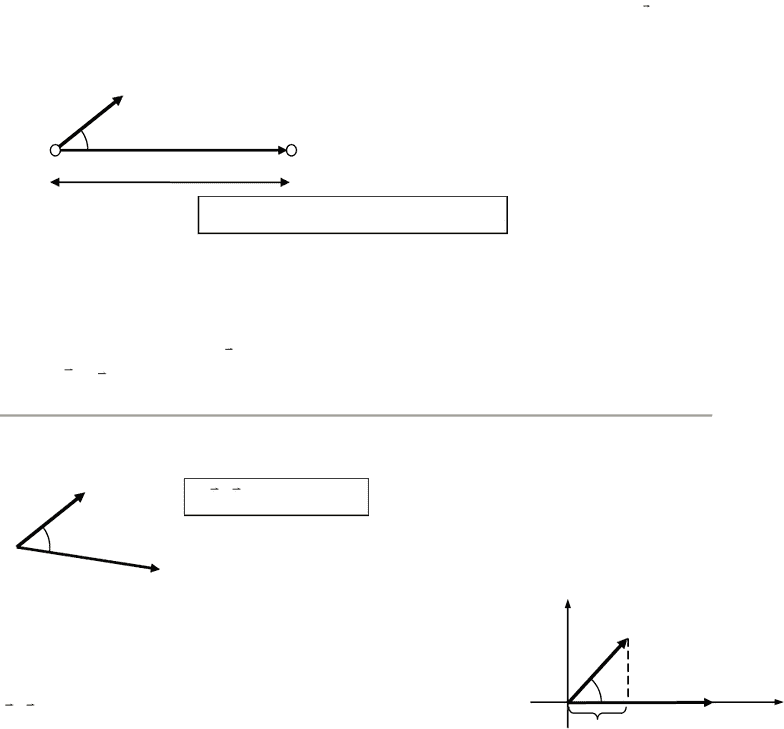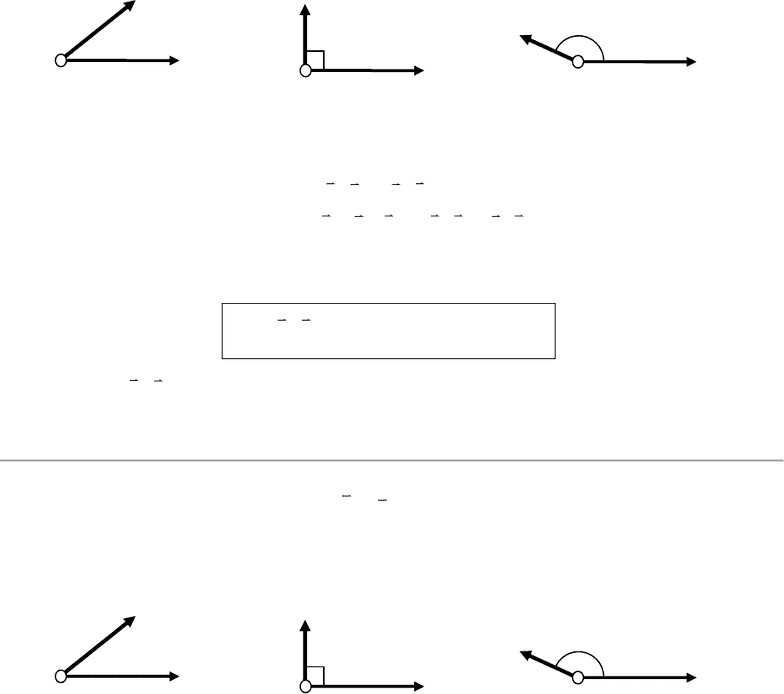Class Notes (1,100,000)
US (480,000)
CU-Boulder (3,000)
PHYS (100)
Marino (5)
Lecture 6

# PHYS 1110 Lecture Notes - Lecture 6: Dot Product, Friction, Chemical Energy

Department
Physics
Course Code
PHYS 1110
Professor
Marino
Lecture
6

This preview shows pages 1-3. to view the full 9 pages of the document.
EW-1
9/28/2013 University of Colorado at Boulder
Work and Energy
Energy is difficult to define because it comes in many different forms. It is hard to find a single
definition which covers all the forms. But we will define energy in the next chapter, as precisely
as possible. For now, let’s just list some forms of energy.
Some types of energy:
kinetic energy (KE) = energy of motion
thermal energy = energy of "atomic jiggling"
potential energy(PE) = stored energy of position/configuration
various kinds of PE:
gravitational
electrostatic
elastic (actually a form of electrostatic PE)
chemical (another form of electrostatic PE)
nuclear
radiant energy = energy of light
mass energy (Einstein says mass is a form of energy.)
Almost all forms of energy on earth can be traced back to the Sun :
Example: Lift a book (gravitational PE) chemical PE in muscles chemical PE in food
cows grass sun (through photosynthesis) !
Some textbooks say that energy is the ability to do work (not a bad definition, but rather vague).
A key idea that we will use over and over again is this: Whenever work is being done, energy
is being changed from one form to another or is being transferred from one body to
another. The amount of work done on a system is the change in energy of the system.
We’ll use the symbol W for work and the symbol U for energy. (We will define work later.) The
English sentence “The work done equals the amount of energy transformed” we can write as
W = U
This is called “The First Law of Thermodynamics”.
[An aside. Actually the First Law of Thermodynamics is this:
“heat added plus work done equals change in energy” or Q + W = U . (Q is the symbol for
heat). In this chapter we won’t consider adding heat to a system (like holding a flame under it),
so Q = 0 and we have just W = U.]
As we'll see later, energy is an extremely useful concept because energy is conserved. When we
say energy is conserved, we mean that energy cannot be created or destroyed; it can only be
transformed from one form to another, or transferred from one body to another. The total amount
of energy everywhere is fixed; all we can do is shuffle it around.

Only pages 1-3 are available for preview. Some parts have been intentionally blurred.EW-2
9/28/2013 University of Colorado at Boulder
Notice that this is not what people normally mean when they say "Conserve energy." When the
power company says "Conserve energy", they really mean "Don't convert the energy stored as
chemical potential energy into other forms of energy too quickly." To a scientist, the phrase
"conserve energy" is meaningless, because energy is always conserved. You can't NOT
conserve energy.
To understand energy and conservation of energy, we must first define some terms: work, kinetic
energy (KE), and potential energy (PE). We’ll get to PE in the next Chapter. Let’s look at work
and KE.
Definition of work done by a force: consider an object moving while a constant force
F
is
applied to the object. While the force is applied, the object moves along some axis (x-axis, say)
through a displacement of magnitude |x| = d.
Notice that the direction of displacement is not the
same as the direction of the force, in general.
Work done by a force F =
F x
W F d F cos d F d ||
F|| = component of force along the direction of displacement, WF = F|| distance
Unit of work: [W] = [F][d] = 1 Nm = 1 joule = 1 J
If the displacement vector is
r
, the work done can be written in terms of the dot product as
F
W F r  
Vector Math interlude:
The dot product of two vectors A and B, "A dot B", is defined as
A B ABcos 
The dot product of two vectors is a number, not a vector. (Later on,
we will see another way to define the product of two vectors, called
the "cross-product". The cross-product of two vectors is a vector.)
The dot product is the magnitude of one vector (say A) times the
component of the other vector (B) along the direction of the first (A).
For instance, suppose that we align the x-axis with vector A. Then
x
A B ABcos AB  
.
F
xi

x| = d
B

B

x
y
Bx
= B cos

Only pages 1-3 are available for preview. Some parts have been intentionally blurred.EW-3
9/28/2013 University of Colorado at Boulder
The dot product is positive, negative, or zero depending on the relative directions of the vectors
A and B. When A and B are at right angles ( = 90o), the dot product is zero. When the angle
is greater then 90o, then the dot product is negative.
It is not difficult to prove, from the definition, that ..
the dot product is commutative:
A B B A  
the dot product is associative:
 
A B C A C B C   
The dot product can be written in terms of the components of the vectors like so:
x x y y z z
A B A B A B A B 
Proof (in 2D) :
 
x y x y x x x y x x y y
ˆ ˆ ˆ ˆ ˆ ˆ ˆ ˆ
A B A i A j B i B j A B i i A B i j ... A B A B    
In the last step, we used the fact that
ˆ ˆ ˆ ˆ
i i 1 and i j=0 
.
So, the work done by a force F is
F
W F r  
. Work is not a vector, but it does have a sign,
(+) or (). Work is positive, negative, or zero, depending on the angle between the force and the
displacement. The formula WF = F d cos gives the correct sign, because cos is negative when
> 90.
Why do we define work this way? Answer: Whenever work is done, energy is being
transformed from one form to another. The amount of work done is the amount of energy
transformed. (This is the First Law of Thermodynamics.)
B

< 90o, AB positive
= 90o, AB = 0
A

> 90o, AB negative
F

r
< 90, W positive
r
= 90, W = 0
F

r
> 90, W negative